JOIN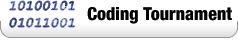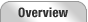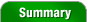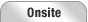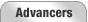Reception Room 1 Room 2 Room 3 Room 4 Final Round Slide ShowPlay-by-Play  |  Photos  |  Video  |  Problem Analysis

## Problem Set Analysis & Opinionby lbackstrom,
TopCoder Member
Friday, December 5, 2003

BalancedTrees
The simplest way to solve this problem is to iterate over heights for a given value of k (the balance factor) and determine the fewest nodes required to make a tree of each height. Since we are dealing with a tree, even when the balance factor is really high, the tree still isn't that tall. If we know the fewest number of nodes required to make a tree of each height, it's easy to find how tall a tree we can make with n nodes. So, the key is to determine the fewest number of nodes to make a tree with a height of h and a balance factor of k. While its a little bit subtle, it isn't too hard to see that to use the fewest nodes we should have a k-balanced tree with height of h-1 for one of the subtrees, and a k-balanced tree of height h-k-1 for the other subtree. The first subtree is sort of a given, since one subtree has to have height h-1, and since less nodes are required for shorter trees, we want to make the other subtree as short as possible, while maintaining the balance constraint. Thus, we can define a recurrence:

```fewest(h,k) = h>0  : 1+fewest(h-1,k)+fewest(h-k-1,k).
h<=0 : 0
```
It turns out that even a naive implementation (without dynamic programming or memoization) will run fast enough:
```	int maxHeight(int k, int n) {
for(int h = 1;;h++){
if(fewest(h,k)>n)return h-1;
}
}
int fewest(int h, int k){
if(h<=0)return 0;
return 1+fewest(h-1,k)+fewest(h-k-1,k);
}
```

BaseballLineup
Most baseball lineups follow a pretty standard format. The fastest player generally leads off, and the power hitters are usually in the 3 and 4 positions. Our simulation wasn't quite advanced enough to take things like speed into account, but it still gives us an idea of how many runs a particular lineup will score. One approach to this is to run a large number of monte carlo simulations. Just do each of the events with the probability given, and take the average of the scores over all simulations. However, this isn't precise enough to work, and usually ends up being off by at least 0.1. To solve the problem in time, and with sufficient precision, we need to use dynamic programming. We need to calculate the probability of achieving each state, in each inning. A state consists of which bases are occupied, who is at bat, how many outs there are, and how many batters there have been in the inning, there are 3*9*2^3*20 = 4320 states. If we start by assigning our start state a probability of 1, we can iteratively compute the probabilities for all of the other states. Basically, we just simulate each event - an out, walk, hit, double, triple, or home run - and that gives us a new state, and we adjust the probability of the new state. So, in high level pseudocode:
```foreach state
foreach event
compute nextState from state and event
probability[nextState] =
probability[nextState]+probability[state]*probability[event]
```
For this to work, we have to iterate the states in the right order, but thats pretty easy to do.

That will give us the probability of the states, but we care about runs. It's actually really simple to compute the expected number of runs, once we know the probabilities of the states. As we are computing the probabilities of the transitions from one state to the next, we can also compute the number of runs expected from the event.
```foreach state
foreach event
compute nextState from state and event
probability[nextState] =
probability[nextState]+probability[state]*probability[event]
runs = runs + probability[state]*probability[event]*runsScored(state,event)
```
The one drawback to this approach is that it leads to a ton of nested loops, and results in some messy code. I usually favor iterative dynamic programming over recursive memoization, but in this case, I think that a memoized solution ends up being cleaner. NGBronson's solution is quite elegant:
```double[][] probs = new double;

public double expectedRuns(String[] stats)
{
for (int i = 0; i < 9; ++i) {
String[] a = stats[i].split(" ");
for (int j = 0; j < 6; ++j) {
probs[i][j] = Integer.parseInt(a[j]) * 0.001;
}
}
return f(0, 0, 0, 20, 1);
}

Map cache = new HashMap();

int[] walkBases = { 1, 3, 3, 7, 5, 7, 7, 7 };
int[] walkRuns = { 0, 0, 0, 0, 0, 0, 0, 1 };
int[] singleBases = { 1, 3, 1, 3, 1, 3, 1, 3 };
int[] singleRuns = { 0, 0, 1, 1, 1, 1, 1, 2 };
int[] doubleRuns = { 0, 1, 1, 2, 1, 2, 2, 3 };

double f(int bases, int batting, int outs, int remaining, int inning) {
if (inning == 10) return 0;
if (outs == 3 || remaining == 0) {
return f(0, batting, 0, 20, inning + 1);
}

String key = bases + " " + batting + " " + outs + " " + remaining + " " + inning;
Double vv = (Double) cache.get(key);
if (vv != null) return vv.doubleValue();

int next = batting + 1;
if (next == 9) next = 0;
double res = 0;

// out
res += probs[batting] * f(bases, next, outs + 1, remaining - 1, inning);

int wb = walkBases[bases];
int wr = walkRuns[bases];
int sb = singleBases[bases];
int sr = singleRuns[bases];
int db = 2;
int dr = doubleRuns[bases];
int tb = 4;
int tr = doubleRuns[bases];
int hb = 0;
int hr = 1 + doubleRuns[bases];

// walk
res += probs[batting] * (wr + f(wb, next, outs, remaining - 1, inning));

// single
res += probs[batting] * (sr + f(sb, next, outs, remaining - 1, inning));

// double
res += probs[batting] * (dr + f(db, next, outs, remaining - 1, inning));

// triple
res += probs[batting] * (tr + f(tb, next, outs, remaining - 1, inning));

// home
res += probs[batting] * (hr + f(hb, next, outs, remaining - 1, inning));

cache.put(key, new Double(res));

return res;
}
```

Nestable
In my opinion, the hard problem was one of the most interesting and most challenging problems that TopCoder has ever put forth. To simplify the discussion, we will call the smallest length of a box x, the next smallest y, and the largest z. It is relatively simple to come up with an O(n^2) implementation: first sort the boxes by x coordinate, and then iterate over the boxes with two nested loops:
```	int[] dp = new int[n];
int ret = 0;
for(int i = 0; i<n; i++){
dp[i] = 1;
for(int j = 0; j<i; j++){
if(nests(i,j))dp[i] = max(dp[i],dp[j]+1);
}
ret = max(ret,dp[i]);
}
```
To make it run faster, we need to use some advanced data structures, TreeSets, or their C++ equivalent. First, we sort by x coordinate in O(n lg n) time. Then, we maintain a large array of TreeSets, where each TreeSet contains the enclosing boxes with the smallest y and z for some number of nestings. For example, Tree1 will contain all of the boxes whose y and z dimensions are not both greater than or equal to the y and z dimensions of some other box in the same set. Once we have these sets, we iterate over all of the boxes, in order by x, and find the highest indexed tree which contains a box that our new box can enclose. We can do this with a binary search over the Trees, with adds a factor of lg(n). Now, for the hard part, quickly determining whether or not a Tree has a box that can be enclosed. To do this, we will impose an ordering on the trees that sorts the boxes by increasing y, and breaking ties by decreasing z. An important observation is that this order will cause the z coordinates to be sorted in descending order, since if they weren't, our tree would contain a box, whose y and z dimensions will both be bigger than some other box in the tree. Now, to determine if a tree contains a box which can be enclosed, we find the box with the largest y coordinate less than the y coordinate of the box that we are adding. This will give us the box with the smallest z coordinate, and thus we can determine if the box we are adding will enclose any boxes in the tree by comparing it to only one box. By using a tree, we can find this box in lg(n) time (java TreeSet.headSet())

So, we can binary search, and find how many nestings a box can be on the outside of in lg2(n) time. Then, we need to insert our box into the appropriate Tree, and maintain the constraint on the Tree so that we can still use the method above. To do this, we can take use the tailSet method, and then just delete all of the boxes that have have bigger y and z dimensions. We also have to be careful to only add the box if it maintains the constraint.

That is the basic algorithm. We have to make a few modifications to deal with ties, but they aren't too tricky. So, in the end our algorithm comes out to be about O(n*lg2(n)), a complexity you rarely see in TopCoder. dgoodman, the writer of this excellent problem, wrote the following:
```import java.util.*;
class Box implements Comparable{
int s,m,b;
Box(int x, int y, int z){
if(x>y){int t=x;x=y;y=t;}
if(y>z){int t=y;y=z;z=t;}
if(x>y){int t=x;x=y;y=t;}
s=x;m=y;b=z;
}
public int compareTo(Object o){
Box q = (Box)o;
if(s!=q.s) return s<q.s ? -1 : 1;
if(m!=q.m) return m<q.m ?  1 : -1;
if(b==q.b) return 0; else return b>q.b ? 1 : -1;
}
}
class Cmp implements Comparator{
public int compare(Object a, Object b){
Box aa = (Box)a, bb = (Box)b;
if(aa.m!=bb.m) return aa.m<bb.m ? -1 : 1;
if(aa.b!=bb.b) return aa.b>bb.b ? -1 : 1;
if(aa.s!=bb.s) return aa.s<bb.s ? -1 : 1;
return 0;
}
public boolean equals(Object a, Object b){ return compare(a,b)==0; }
}
public class Nestable{
int dumCount(int a, int p, int n){
int length=1; Box[] bx = new Box[n];
for(int i=0; i<n; i++){
length = (length*a)%p; int x=length;
length = (length*a)%p; int y=length;
length = (length*a)%p; int z=length;
bx[i]= new Box(x,y,z);
}
Arrays.sort(bx);  // lexico   s,m,b
int[] ct = new int[n];  //num boxes it nests inside itself
int mx=0;
for(int i=0;i<n;i++){
ct[i] = 0;
for(int j=0;j<i;j++)if(ct[j]>=ct[i]){
if(bx[i].m>bx[j].m && bx[i].b>bx[j].b && bx[i].s>bx[j].s){
ct[i]=ct[j]+1; if(ct[i]>mx) mx=ct[i];
}
}
}
return mx+1;
}
public int maxCount(int a, int p, int n){
if(1==2) return dumCount(a,p,n);
int length=1; Box[] bx = new Box[n];
for(int i=0; i<n; i++){
length = (length*a)%p; int x=length;
length = (length*a)%p; int y=length;
length = (length*a)%p; int z=length;
bx[i]= new Box(x,y,z);
}
Arrays.sort(bx);  // lexico   s,m,b

TreeSet[] list = new TreeSet[n]; int nf=0;  //frontiers
Cmp cmp = new Cmp();
for(int i=0;i<bx.length;i++){
Box b = bx[i];
if(nf==0 || greater(b,list[nf-1])){
TreeSet newF = new TreeSet(cmp); newF.add(b);
list[nf++]=newF; continue;
}
int lo = 0, hi=nf-1;  //fits in lo..hi at most ie grtr[lo-1],!grtr[hi-1
while(lo<hi){
int middle = (lo+hi)/2;
if( greater(b, list[middle]) ) lo=middle+1; else hi=middle;
}
insert(list[lo],b);
}
return nf;
}
void insert(SortedSet f, Box b){
SortedSet tail = f.tailSet(b);
Iterator it = tail.iterator(); it.next();
while(it.hasNext()){
Box x = (Box)it.next(); if(x.b<b.b) return;
it.remove();
}
}
boolean greater(Box b, SortedSet s){
while(true){
if(prev.b>=b.b) return false;
if(prev.s != b.s) return true;
}
}
int gcd(int a, int b){ return b==0 ? a : gcd(b,a%b); }
boolean div(int a, int p){ //is a power of a divis by p?
int g = gcd(a,p);
while(g>1){ p=p/g; g=gcd(a,p); }
return p==1;
}
}
```